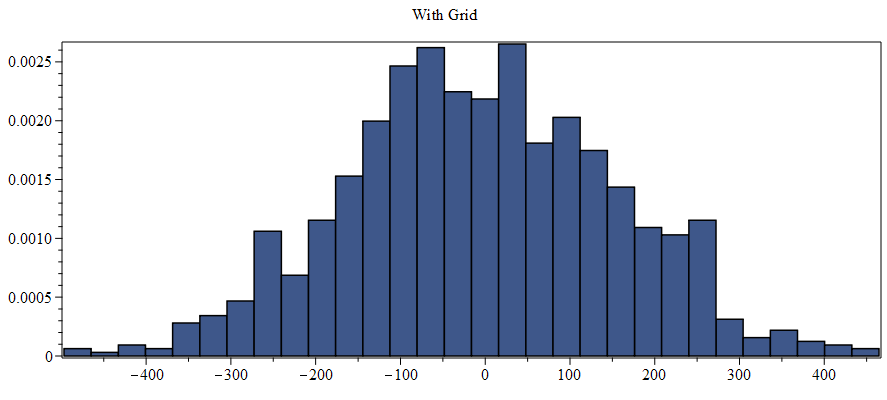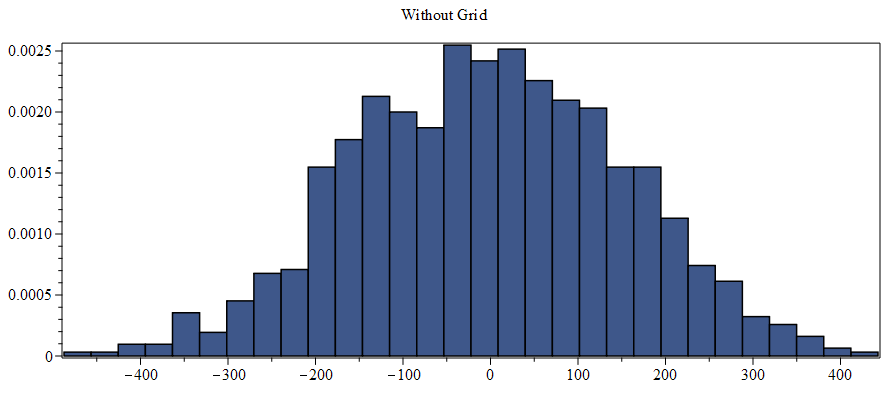:

## Using Statistics:-Sample in the `Grid` environment

Maple 2019

I experienced a significant obstacle while trying to generate independent random samples with Statistics:-Sample on different nodes of a Grid multi-processing environment. After many hours of trial-and-error, I discovered an astonishing workaround, and I achieved excellent time and memory performance. Since this seems like a generally useful computation, I thought that it was worthy of a Post.

This Post is also worth reading to learn how to use Grid when you need to initialize a substantial environment on each node before using Grid:-Map or Grid:-Seq.

All remaining details are in the following worksheet.

How to use Statistics:-Sample in the `Grid` environment

Author: Carl Love <carl.j.love@gmail.com> 1 August 2019

I experienced a significant obstacle while trying to generate indenpendent random samples with Statistics:-Sample on the nodes of a multi-processor Grid (on a single computer). After several hours of trial-and-error, I discovered that two things are necessary to do this:

 1 The random number generator needs to be seeded differently in each node. (The reason for this is easy to understand.)
 2 The random variables generated by Statistics:-RandomVariable need to have different names in each node. This one is mind-boggling to me. Afterall, each node has its own kernel and so its own memory It's as if the names of random variables are stored in a disk file which all kernels access. And also the generator has been seeded differently in each node.

Once these things were done, the time and memory performance of the computation were excellent.

 > restart :
 > Digits:= 15 :
 > #Specify the size of the computation: (n1,n2,n3):= (100, 100, 1000): # n1 = size of each random sample; # n2 = number of samples in a batch; # n3 = number of batches.
 > # #Procedure to initialize needed globals on each node: Init:= proc(n::posint) local node:= Grid:-MyNode();    #This is wrapped in parse so that it'll act globally. Otherwise, an environment    #variable would be reset when this procedure ends.    parse("Digits:= 15;", 'statement');    randomize(randomize()+node); #Initialize independent RNG for this node.    #If repeatability of results is desired, remove the inner randomize().    (:-X,:-Y):= Array(1..n, 'datatype'= 'hfloat') \$ 2;    #Perhaps due to some oversight in the design of Statistics, it seems necessary that    #r.v.s in different nodes **need different names** in order to be independent:    N||node:= Statistics:-RandomVariable('Normal'(0,1));    :-TRS:= (X::rtable)-> Statistics:-Sample(N||node, X);    #To verify that different names are needed, change N||node to N in both lines.    #Doing so, each node will generate identical samples!    #Perform some computation. For the pedagogical purpose of this worksheet, all that    #matters is that it's some numeric computation on some Arrays of random Samples.    :-GG:= (X::Array, Y::Array)->       evalhf(          proc(X::Array, Y::Array, n::posint)          local s, k, S:= 0, p:= 2*Pi;             for k to n do                s:= sin(p*X[k]);                  S:= S + X[k]^2*cos(p*Y[k])/sqrt(2-sin(s)) + Y[k]^2*s             od          end proc          (X, Y, n)       )          ;    #Perform a batch of the above computations, and somehow numerically consolidate the    #results. Once again, pedagogically it doesn't matter how they're consolidated.      :-TRX1:= (n::posint)-> add(GG(TRS(X), TRS(Y)), 1..n);        #It doesn't matter much what's returned. Returning `node` lets us verify that we're    #actually running this on a grid.    return node end proc :

The procedure Init above uses the :- syntax to set variables globally for each node. The variables set are X, Y, N||node, TRS, GG, and TRX1. Names constructed by concatenation, such as N||node, are always global, so :- isn't needed for those.

 > # #Time the initialization: st:= time[real]():    #Send Init to each node, but don't run it yet:    Grid:-Set(Init)    ;    #Run Init on each node:    Nodes:= Grid:-Run(Init, [n1], 'wait'); time__init_Grid:= time[real]() - st;The only purpose of array Nodes is that it lets us count the nodes, and it lets us verify that Grid:-MyNode() returned a different value on each node.

 > num_nodes:= numelems(Nodes);> #Time the actual execution: st:= time[real]():    R1:= [Grid:-Seq['tasksize'= iquo(n3, num_nodes)](TRX1(k), k= [n2 \$ n3])]: time__run_Grid:= time[real]() - st> #Just for comparison, run it sequentially: st:= time[real]():    Init(n1): time__init_noGrid:= time[real]() - st; st:= time[real]():    R2:= [seq(TRX1(k), k= [n2 \$ n3])]: time__run_noGrid:= time[real]() - st;R1 and R2 will be different because different random numbers were used, but they should have similar histograms.

 > plots:-display(    Statistics:-Histogram~(       , #side-by-side plots       'title'=~ <<"With Grid\n"> | <"Without Grid\n">>,       'gridlines'= false    ) );

(Plot output deleted because MaplePrimes cannot handle side-by-side plots!)

They look similar enough to me!

Let's try to quantify the benefit of using Grid:

 > speedup_factor:= time__run_noGrid / time__run_Grid;Express that as a fraction of the theoretical maximum speedup:

 > efficiency:= speedup_factor / num_nodes;I think that that's really good!

The memory usage of this code is insignificant, which can be verified from an external memory monitor such as Winodws Task Manager. It's just a little bit more than that needed to start a kernel on each node. It's also possible to measure the memory usage programmatically. Doing so for a Grid:-Seq computation is a little bit beyond the scope of this worksheet.

 >

Download GridRandSample.mw

Here are the histograms:﻿